﻿ Second law Analysis of Gas TurbinesSecond law Analysis of Gas Turbines

 CONTENTS 12pg 45K 11fig 1. Abstract 2. Background 3. Focus 4. The plural energy system 5. Energy vs work and heat 6. Thermal temperature and chemical temperature 7. Splitting up the exergy concept 8. The carnot ratio and helmholtz ratio 9. Polytropic gas turbine analysis 10. Six open cycle gas turbines analyzed 11. Inputs for metal 49MW 4Pr gas turbine 12. Compressor 13. Heat exchanger 14. Turbine 15. Combustion 16. Bottoming cycle and exhaust 17. Efficiency of total cycle 18. Reduction of combustion irreversibility's 19. Conclusions 20. References 21. Symbols 22. Notes 23. Revision history >>>Copyright 2008Aug11 by Ben Wiens...energy scientist 1. ABSTRACT     In this paper gas turbines are analyzed in a reasonably uncomplicated way. Concepts are targeted at those having a basic science and engineering knowledge as well as those with advanced degrees. The second law analysis methods presented use simple formulas and concepts. Even so, they are more detailed than a typical second law evaluation. No understanding of entropy is required as basic concepts revolve around the helmholtz ratio. A complete set of simple formulas for analyzing a gas turbine are provided. The equations can also be downloaded as a MathCAD file universalgt.mcd. These formulas adjust for a multitude of varying conditions such as cooling bypass, pressure ratio, and pressure losses. Four popular gas turbines and two proposed designs are analyzed in detail. Addition of heat exchanger and fuel cells are discussed. 2. BACKGROUND     In a typical engineering second law evaluation, the amount of exergy that is retained in a system is calculated. In these methods there is considered to be an amount of energy as well as exergy residing in a system. Exergy is the amount of useful work that can be drawn from this energy based on a certain ambient temperature of the environment. Exergy ...is not considered a form of energy but a designation of the quality of energy (Moran 1994). During each small part of a process, there are losses of this exergy. These can be itemized in a table but are often shown as bar charts. It is easy to see what the exergy loss of each separate processes is and this leads to an understanding of how to improve the efficiency of the system. Second law evaluation methods are well developed presently (Horlock 1992). Several current books have been written on these methods (Ahern 1980, Moran 1982). These methods are not new, for example Professor Goodenough in the US included methods of availability analysis in his textbooks in 1911 (El-Sayed 1989). Recently however there have even been heated debates over exergy fundamentals at American Society of Mechanical Engineers sanctioned conferences (Moran 1994). 3. FOCUS     The second law analysis methods proposed in this web-booklet are not based on what I call the singular energy system using energy and exergy just described. Instead a plural energy system is used . Seventeen concepts identified with (C1) etc. will be introduced that do not appear to be included in a typical gas turbine second law analysis. The first focus is to introduce tools that will make the second law analysis more detailed. The next focus is to present a system that hopefully is simpler to use by professionals without an extensive background in advanced energy concepts. Only simple equations and English character symbols are used throughout.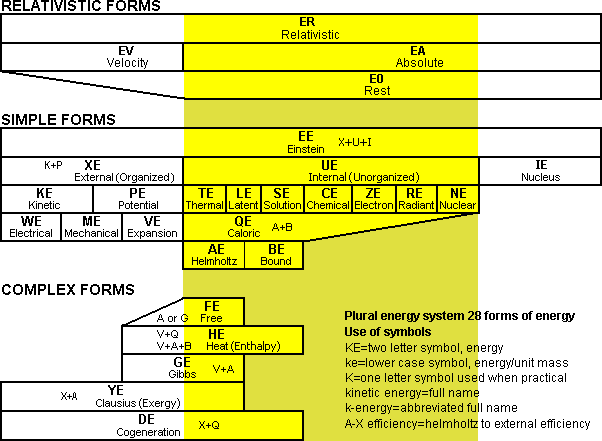Fig 1 Bar chart illustrating the plural energy system     The first concept that will be introduced is the plural energy system as shown in Fig 1. Such a system was started in the 1800s by Gibbs and especially Helmholtz who it appears also viewed these terms as real subforms of energy (Bailyn 1994). In this system, the concept of exergy is replaced by clausius energy ...is a specific form of energy composed of external energy and helmholtz energy. There are 28 forms of energy shown in total. A key advantage of the plural energy system is that it allows the present exergy evaluation to be broken into eleven divisions; external, kinetic, potential, electrical, mechanical, expansion, helmholtz, free, gibbs, clausius, and bound energy. This makes a second law evaluation more precise as each of these are not really equal in worth when comparing realistic processes.     At the head of the center chart titled "simple forms" is einstein energy. This is the most generic form of energy which all other forms of energy can be thought to be made of. This is the only form of energy that can not be destroyed. This energy can be logically split up into three forms, external energy, internal energy, and nucleus energy.     Nucleus energy is that energy contained inside particles that can not typically be utilized or extracted such as the energy contained in the nucleus of atoms.     The unorganized energy contained in particles the size of molecules and smaller is called internal energy. It can be further subdivided into thermal, latent, solution, chemical, electron, nuclear, and radiant energy forms.     When a larger object such as a cannonball is shot out of a cannon, it contains energy that is traveling in many different directions, however there is now a net linear direction of a collection of particles. The amount of this organized energy that can be considered to have a net direction is called external energy. It can be split up further into the basic forms of kinetic and potential or other more explicit forms.     Expansion energy is a special hybrid type of external energy stored in expanding systems of molecules that behaves partly like internal energy. Some forms of radiant energy such as black body radiation behave according to internal energy laws while forms such as radio waves behave according to external energy laws. Caloric energy, a new term, represents the amount of internal energy that will flow between two reservoirs. Helmholtz himself suggested that this be divided up (Bailyn 1994) between free energy, which today is more specifically renamed helmholtz energy, and bound energy.     Complex forms of energy are those that contain a mixture of internal and external energy. Heat energy also known as enthalpy is composed of expansion energy and caloric energy. Gibbs energy is composed of helmholtz energy plus expansion energy. Clausius energy is composed of external energy and helmholtz energy. Cogeneration energy is composed of external energy plus caloric energy. 5. ENERGY vs WORK AND HEAT (C2)     In engineering, the terms work and heat are widely used in thermodynamics. These terms are very limiting when working with more complex energy calculations. It is better to use energy terms entirely. In currency and accounting systems there is only one form of money stated. Money is either in an account or money is being transferred. In energy systems we can also say there is so much energy at a certain point of the process or that energy is transferred during a process. It is also more explicit to say that helmholtz energy was converted to external energy than mentioning that work was done or heat was transferred. The term external energy is introduced to replace the need for using the term work. The concept of heat transfer can be replaced with the broader internal energy transfer which can take seven different forms. 6. THERMAL TEMPERATURE AND CHEMICAL TEMPERATURE (C3)     Temperature ..is a scale that is proportional to the average thermal energy contained in individual molecules. Thermal energy is the motion energy of the molecules. When an exothermic chemical reaction occurs, the excess energy produced is not initially dribbled out in randomly sized quantities of energy. Rather one virtual photon is produced for each new molecule produced. This virtual photon preserves the helmholtz energy produced in the reaction by storing the largest amount of energy possible in each virtual photon. Chemical temperature ..is a new term that can be used to describe the energy contained in virtual photons for chemical energy with units of Kelvin  and symbol T just like temperature. To avoid confusion we can use the term thermal temperature instead of just temperature.In the singular energy system, all more specific forms of clausius energy are generally lumped together. The typical exergy equation (1) does start with five different types of energy in the equation but then adds them together.     In general when it is stated that a certain gas turbine had an exergy efficiency of 85% it refers to the percentage of clausius energy in an amount of caloric energy that was consumed using the terms from Fig 1. In the plural energy system, the efficiency would be stated based on the already defined energy terms as shown in Fig 1. A gas turbine powerplant could have a fuel A-X efficiency, in other words a helmholtz energy in the fuel to external energy efficiency. Other useful terms would be Q-X Eff, Q-Y Eff, G-Y Eff and the list goes on. These are simply called second law efficiencies.     It is desirable when possible to calculate helmholtz energy, expansion energy, external energy, and bound energy separately. These four forms of energy can be combined to form the others desired. Figure 2 shows the typical efficiency of converting different forms of energy to external energy (X-energy) in an advanced gas turbine.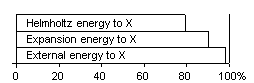Fig 2 Typical efficiency of conversion to X-energy in GT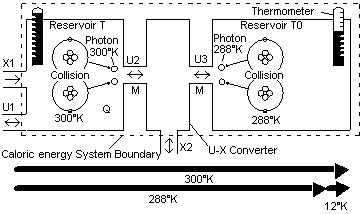Fig 3 System diagram for conversion of energy     Entropy is used extensively in a typical exergy evaluation to calculate the bound energy produced. In Eq (1) this is the TS term. As can be seen, the exergy equation is an additive-subtractive equation.     In the helmholtz ratio method (C5) proposed, the use of entropy is not always necessary. A complete gas turbine will be evaluated later without using entropy. When entropy is required in more complex calculations, it is often better to first calculate the helmholtz ratio. This ratio is more versatile than the subtractive process.     A useful tool (C6) in analyzing any conversion involving internal energy is the system diagram as shown in Fig 3. The system shown is similar to that listed in common textbooks with the following exception. The system boundary is drawn around both reservoirs. This is possible due to the introduction of caloric energy, which is a function of two reservoirs. Note that the T0 reservoir must always be considered infinitely larger than the T reservoir for the helmholtz ratio or entropy.     The ratio of external energy X2 that could be extracted at any instantaneous moment to the caloric energy that would flow is equal to the carnot ratio Cr. The carnot ratio can be plotted on a graph as shown in Fig 4. Based on a fixed ambient temperature T0, a single curve can be drawn. This is a useful tool (C7) for analyzing any conversion process because it shows what the changing conversion efficiency will be during the process.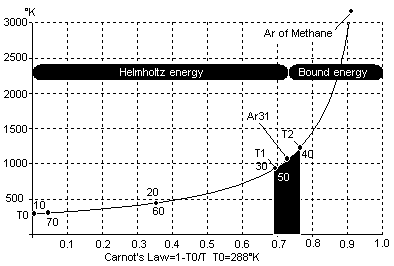Fig 4 Carnot ratio diagram for Metal 4Pr of Fig 6     Because many processes occur over varying temperatures, it is helmholtz energy A and the helmholtz ratio Ar that is mostly used. It is important to think of helmholtz energy A and bound energy B in the right way. The helmholtz energy A is the part of caloric energy Q that could be converted into external energy X in a future process. The bound energy B is the caloric energy Q that could have been converted into external energy X if the conversion had started at an infinite temperature and progressed to the present point.     In Fig 3, if an amount of external energy X2 is transferred into the system by means of the U-X converter, it can be reversibly stored at many different temperatures of Reservoir T. If an amount of external energy X1 is transferred into the system by frictional means into a single reservoir, it must be converted into caloric energy at an infinite temperature (theoretically possible) to be reversibly stored in the system.     These relationships are valid both for thermal energy as well as for other forms of internal energy. It is however often hard to determine what the temperature of a chemical bond would be and so the helmholtz energy or gibbs energy is often calculated from tables.     Simple equations can often be used to calculate the helmholtz ratio. For flows of internal energy that will occur at constant temperatures, Eq (2) which is basically Carnot's Law can be used. For variable temperature processes of constant specific heat such as perfect gases Eq (3) can be used. For variable temperature process of variable specific heat such as steam processes entropy is used with Eq (4 plus 5).9. POLYTROPIC CYCLE GAS TURBINE ANALYSIS (C8)     It is necessary to calculate the realistic efficiency of a gas turbine because there is no way of knowing from the basic theory which cycle will result in the highest efficiency. This is because of the various inefficiencies of the components involved. Gas turbines are often modeled using perfect gas calculations. The advantage is that cycle calculations can be done easily on a computer using simple equations.     The realistic efficiencies of all gas turbines in this web-booklet have been modeled with polytropic inefficient processes. In a polytropic compression process part of the thermal energy flows out of the system. In an inefficient process part of the expansion energy is converted into thermal energy during the process. Variable specific heats of combustion gases are simulated by additions of thermal energy in the turbine rather than just additions of thermal energy in the combustion chamber. Compound cycles using intercoolers and reheat are modeled by different gas exponents. In this way one set of equations can be used for all different types of gas turbines. This makes it easy to evaluate radically different designs quickly. The helmholtz ratio is ideal for dealing with these polytropic processes. 10. SIX OPEN CYCLE GAS TURBINES ANALYZED (C9)     In Table 1, six gas turbines were analyzed. Four are commercially available and two are proposed designs. Manufacturers do not readily give out specific information about their gas turbines. The different figures have been pieced together from various sources. Both the final efficiency and the known inputs match quite closely. All 6 gas turbines were evaluated with the same formulas, however compressor and turbine efficiencies and pressure losses were altered for all gas turbines to fall in line with the type of engine.     The Solar Saturn is a simple cycle gas turbine with 6.5 pressure ratio Pr and a non cooled turbine. The Westinghouse/Rolls Royce WR-21 is a heat exchanged gas turbine designed specifically to have good part load efficiency on fast ships. The General Electric S-160 combined cycle uses an aeroderivative engine. Its high 29.5Pr results in a severe amount of compressor and turbine B-energy produced at sea level. Its steam bottoming cycle is not as efficient as in larger powerplants. The Siemens V84.3A represents about the best combined cycle technology available presently. The 16.6Pr of this engine can be shown to result in optimal efficiency for a combined cycle. The Ceramic 4Pr and the Metal 4Pr are proposed low pressure heat exchanged gas turbines which can be used with an optional combined cycle. They are two favorite gas turbines that emerged after evaluating 100s of radically different designs. The Ceramic 4Pr is a gas turbine which may some day be practical. The Metal 4Pr gas turbine operates at 1223°K which means that no cooling at all is required. An acid resistant boiler is required though because of the low boiler exhaust temperatures.     In the next pages the 52 formulas used to calculate the gas turbine efficiencies in Table 1 are shown, a lot for a short article. They are included because it allows those interested a chance to quickly evaluate the helmholtz ratio method and the particular equations suggested by typing them into an off the shelf Math computer program (C9). 11. INPUTS FOR METAL 49MW 4PR GAS TURBINE     All the following equations are oriented to open cycle gas turbines. Not all equations are listed in the order of calculation. All temperatures are in Kelvin. Natural gas is the fuel used. Inputs must abide by the following T40>T20, n11>1.0, n41>1.012. COMPRESSOR (C10, 11, 12)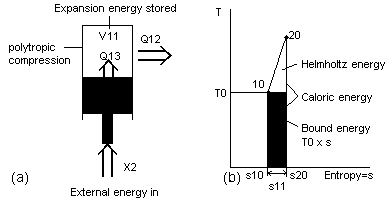Fig 5 Differences between ratio and subtractive calculations     To model the gas turbine compression process, a piston in a cylinder is used as shown in Fig 5a. The compression is an inefficient polytropic process which would ideally model the intercooled compressor of the WR-21 powerplant. In the proposed method of analyzing such a process, the external energy X2 that is supplied to the system is converted into three new separate streams of energy. There is expansion energy V11 which is stored inside the cylinder, caloric energy Q13 due to friction which is also stored in the cylinder and caloric energy Q12 that is created due to transfer out of the cylinder.     If such a process is calculated with entropy and the subtractive process as shown in Eq (1) it would be found that at the end of the compression process the helmholtz energy A in the two streams of caloric energy Q12 and Q13 would be combined into one amount. This is illustrated with the T-S diagram in Fig 5b. Only one amount of caloric energy Q and one amount of bound energy B are possible for an individual process. The helmholtz ratio however can be multiplied by the caloric energy Q of each stream of energy by using a variation of Eq (6).     Because the modern gas turbine operates as a continuous process, the compression process is required to create a pressure differential so that external energy X can be extracted in a gas expansion. In the simple cycle gas turbine the pressure ratios need to be high to be able to extract as much external energy X in one expansion as possible. The combined cycle can utilize the thermal energy T in the exhaust but the steam turbine is slightly less efficient than the gas turbine so a high pressure ratio is required as well. The simple and combined cycle also need high pressure-ratios to elevate the temperature of the air before combustion for efficient operation. The compression process increases the temperature of the air in an adiabatic or reversible fashion. The higher the compressor exit temperature the less bound energy B will be produced during the even higher temperature combustion process. Unfortunately as the gas turbine's combustion becomes more efficient at higher combustion chamber inlet temperatures, the amount of net work from the gas turbine decreases as the compressor work becomes the same as the turbine work. In modern simple and combined high temperature industrial gas turbines, efficiency peaks at around Pr=17.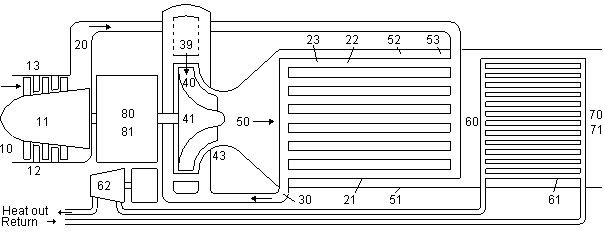Fig 6 Gas turbine model used in Table 1

 Ceramic4Pr Metal4Pr Siemens V84.3A GE S-160 WR-21 Solar Saturn Inputs ex=exchanged comb=combined cycle Symbol 49MWex, comb 49MWex, comb 250MWcomb 53MWcomb 25MWex 1.08MWsimple Nominal compressor efficiency at Pr=2 Eff11n 0.92 0.92 0.90 0.92 0.88 0.86 Nominal turbine efficiency at Pr=2 Eff41n 0.92 0.92 0.90 0.92 0.88 0.96 Compressor exponent n11 1.39 1.39 1.39 1.39 1.24 1.39 Turbine exponent n41 1.25 1.30 1.33 1.37 1.33 1.30 Compressor inlet temperature T10 288°K 288°K 288°K 288°K 288°K 288°K Combustion exit temperature T39 1973°K 1223°K 1623°K 1623°K 1437°K 1100°K Boiler exit temperature T70 348°K 348°K 348°K 422°K 348°K 348°K Compressor pressure ratio Pr 4.0 4.0 16.6 29.5 14.0 6.5 Exchanger high pressure loss L21 0.035 0.035 - - 0.10 - Combustor pressure loss L31 0.05 0.05 0.05 0.05 0.05 0.05 Exchanger low pressure loss L51 0.02 0.02 0 0 0.05 0 Boiler low pressure loss L61 0.02 0.02 0.02 0.02 0 0 Exchanger effectiveness at equal flow Eff21 0.975 0.95 - - 0.88 - Bottoming conversion of exhaust A-energy Eff62 0.71 0.71 0.71 0.60 - - Air cooling bypass % of compressor By41 0 0.00 0.15 0.10 0.15 0 Alternator efficiency Eff81 0.99 0.99 0.99 0.99 0.99 0.95 Calculations - - - - - - - Adjusted compressor Eff due to Pr and size Eff11 0.912 0.912 0.878 0.844 0.845 0.845 Compressor exit temperature T20 441°K 441°K 706°K 887°K 527°K 536°K Equivalent turbine inlet temperature no cooling T40 1973°K 1223°K 1486°K 1549°K 1301°K 1100°K Adjusted turbine efficiency due to Pr and size Eff41 0.904 0.912 0.853 0.827 0.820 0.830 Turbine exit temperature T50 1549°K 925°K 809°K 720°K 757°K 765°K Adjusted heat exchanger effectiveness Eff22 0.975 0.950 - - 0.748 - Total internal energy added u80 637.9 395.8 893.0 722.2 670.0 650.0 External energy from bottoming x62 25.4 24.4 157.7 89.9 - - Total external energy extracted x82 478.8 239.3 519.4 367.2 278.8 166.6 Helmholtz ratio - - - - - - - Compressor Ar11 19.8% 19.8% 38.2% 45.9% 27.2% 27.9% Combustion of fuel Ar31 83.4% 72.7% 72.5% 75.7% 70.8% 63.3% Turbine Ar41 83.6% 73.0% 74.1% 73.4% 71.3% 68.8% Exchanger out Ar22 68.1% 57.0% - - 55.7% - Exchanger in Ar52 67.0% 55.3% - - 53.7% - Boiler Ar61 29.0% 28.7% 47.3% 48.4% 35.0% 45.6% Exhaust Ar71 9.2% 9.2% 9.2% 17.9% 9.2% 9.20% A-B-energy loss % of fuel A-energy - - - - - - - Compressor friction b13 1.73% 2.79% 3.60% 7.16% 6.04% 4.35% Exchanger slippage b21 1.94% 1.97% 0 0 0.61% 0 Exchanger high pressure b23 0.11% 0.18% 0 0 0.09% 0 Combustion b31 16.48% 27.18% 27.06% 24.10% 28.90% 35.85% Turbine friction b43 1.51% 2.44% 3.14% 6.00% 4.99% 3.67% Combustion pressure drop b44 0.16% 0.26% 0.03% 0.02% 0.04% 0.09% Exchanger low pressure b53 0.25% 0.40% 0 0 0.61% 0 Bottoming cycle or exhaust loss no bottoming b62 1.62% 2.52% 7.21% 8.30% 15.70% 29.55% Boiler pressure drop b61 0.26% 0.41% 0.12% 0.23% 0 0 Exhaust a71 0.87% 1.40% 0.62% 3.33% 0.84% 0.85% Generator b82 0.75% 0.60% 0.58% 0.51% 0.42% 1.28% Powerplant fuel A-X efficiency - 74.30% 59.84% 57.58% 50.34% 41.76% 24.35% Cogeneration fuel A-Y efficiency - 75.17% 61.23% 58.20% 53.67% 58.14% 53.27%

 Table 1 Comparison of six gas turbines     One of the problems with higher pressure ratios is that the compressor and turbine polytropic efficiency becomes less (C10). Equation (18, 37) adjust for this and are plotted in Fig 7. Single spool engines like the Siemens V84.3A are most affected and multi-spool like the GE S-160 are less affected (Wilson 1984).     In the heat exchanged gas turbine, high pressure ratios are not necessary. In the same way that the compressor mechanically increases the temperature of the molecules, the heat exchanger does so by a thermal method. Heat exchanging appears to be a more efficient method. Figure 8 shows that the higher pressure ratio Siemens gas turbine is 82.7% efficient in converting A-energy to X-energy while the Metal 4Pr heat exchanged engine is 87.1% efficient even when the heat exchanger losses are factored in (C11). While the heat exchanger largely recycles A-energy through the engine, the simple and combined cycle engines recycle more valuable X-energy through the system. While the A-energy in the heat-exchanger is converted at up to 98% A-A efficiency, the simple cycle recycles expansion energy through the system at a lower 90% V-V efficiency.     Calculations showed that the Metal 4Pr heat exchanged combined cycle powerplant was more efficient than a complex compounded 256Pr high pressure gas turbine with 4 stages of intercooling and reheat, heat exchanging and bottoming cycle. Both used the same turbine inlet temperature. The complex powerplant's 2nd, 3rd and 4th stages produced as little as 92% of the work as the 1st because the compressors operate at elevated temperatures due to imperfect intercooling. So even though the Metal 4Pr had greater heat exchanger losses than the complex powerplant, its higher work output more than offset these losses. (C12)     When external energy X is converted to caloric energy Q through friction in the compressor or turbine, it is not all converted to bound energy B. In the S-160 the Ar11=46% which is calculated by Eq (23). This means almost half of the external energy X converted into caloric energy Q is retained as helmholtz energy A. The helmholtz ratio Ar is even higher in the turbine.13. HEAT EXCHANGER (C13, 14)Fig 7 X-V efficiencies with increasing pressure ratios     Not only can the heat exchanger reduce bound energy B production in the combustion chamber, it is also able to recycle much of the thermal energy T in the exhaust to the incoming air. There is a limit to how much of the exhaust can be recycled because of the compressor exit temperature. The compressor can be intercooled, but calculations showed that using a bottoming cycle steam turbine instead of one intercooling stage on the Metal 4Pr resulted in higher efficiencies in large powerplants.     The effectiveness of a heat exchanger is the amount of thermal energy T transferred through a certain temperature range compared to how much ideally should have been transferred. The loss of effectiveness is due to temperature differences due to imperfect heat transfer. This does not result in a loss of thermal energy T, just more thermal energy T is passed out to the boiler or exhaust. The Metal 4Pr lists a 95% effective heat exchanger which seems high compared to the WR-21 heat exchanger of 88%. If however the WR-21 heat exchanger is analyzed, it has a 22°K temperature differential between exhaust and incoming gases which is identical to the Metal 4Pr. This is because the Metal 4Pr engine has a 460°K drop in temperature through the heat exchanger while the WR-21 appears to only have a 175°K drop (C13).     In many heat exchanged gas turbines the specific heat of the exhaust gases is higher than the incoming air and this can result in a reduced effectiveness (El-Masri 1987). Calculations however showed that when the methane fuel is passed through the heat exchanger as well, the exhaust had a lower specific heat than the incoming air. This would result in increased effectiveness. Air cooled turbines need to bypass some of the compressor air to cool the turbine. This reduces the effectiveness as well because of imbalances in the flow and is adjusted with Eq (27). In the WR-21 this reduced the effectiveness from a nominal 88% down to only 75% (C14).     Due to the differential temperatures there is a loss of helmholtz energy A in the heat exchanger, here these are called slip losses. This can be rated as an A-A efficiency. The bound energy B created is as a result of a certain amount of helmholtz energy A being available on one side, and a lesser amount being available on the other. This is calculated by Eq (33). Pressure losses calculated in Eq (34, 35, 47, 52) use part of the typical entropy equation as only one type of loss is being considered.14. TURBINE (C15)Fig 8 A-X efficiency in 3 gas turbines of Table 1     In a heat exchanged gas turbine the hot gases are only expanded slightly before the remaining thermal energy T is recycled to the incoming gases. This would seem wasteful, however maintaining a high temperature in the turbine results in a high ratio of turbine to compressor work and results in a higher A-X efficiency of the combined compressor and turbine unit.     The biggest problem with the turbine is that it is exposed to hot combustion gases. Air cooling of modern gas turbines is usually employed. This cooling air creates a turbulence. There is also a small pressure loss even when multi-pressure cooling is used. The cooling air and turbine blades reduce the mean turbine temperatures. All these losses resulted in a S-160 turbine X-V efficiency of only 82.7%. If a high temperature low pressure turbine were cooled, nearly all the stages would require cooling. Up to 30% of the compressor air would need to be diverted. The reduction in efficiency is estimated with Eq (36, 37). It appears that air cooling of the turbine is not compatible with a heat exchanged gas turbine (C15). The Metal 4Pr was rather designed to run at low temperatures without cooling and still have a high efficiency.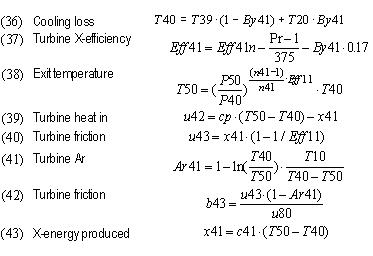15. COMBUSTIONFig 9 Fuel A-energy to X-energy in Siemens V84.3A     It is well known that the single largest production of bound energy B in the gas turbine occurs due to necessary cooling of gases in the combustion chamber and first stages of the turbine. For an advanced combined cycle such as the Siemens V84.3A, the compressor, turbine, bottoming cycle and exhaust bound energy B created is small compared to this cooling bound energy B created. This is illustrated in Fig 9. Production of bound energy B in the combustion chamber occurs because the chemical energy C has been converted to thermal energy T at a lower temperature than the maximum possible for the particular fuel used. Methane has a helmholtz ratio of 91.9% based on the higher heating value (hhv). If this is plotted on the carnot ratio diagram of Fig 4, this equals a mean temperature in Reservoir T of 3556°K, based on a 288°K ambient temperature. In the Siemens V84.3A the mean temperature of combustion however is only 1048°K which equals a helmholtz ratio Ar of 72.5%. The bound energy B produced by this difference in helmholtz ratios Ar is calculated with Eq (48). Note that in Table 1 the cooling losses of the turbine are approximated by creating an adjusted maximum combustion temperature in Eq (36).     In North America, gas turbine efficiency is based on the lower heating value (lhv) in the fuel. This lhv is nearly the same as the gibbs G or helmholtz energy A of the fuel so in Table 1 Ar32=1.00 was used for the fuel and the more precise A-X efficiency is calculated.     It is a higher mean combustion temperature that is desired, not just a higher peak combustion temperature. Low pressure, simple, and combined cycle gas turbines have a low combustion inlet temperature which brings down the mean temperature. For example in Table 1, the Solar Saturn with 6.5Pr has a combustion inlet temperature of only 536°K. From the graph in Fig 4 this would result in a Cr=46%. Over half of the caloric energy Q would become bound energy B at the start of combustion. In the Ceramic 4Pr engine both the peak combustion temperature as well as the combustion chamber inlet temperature is high. This results in an Ar31=83.4%. This is +10.6% from that of the Metal 4Pr.16. BOTTOMING CYCLE AND EXHAUST (C16)     Calculations show that the best large modern triple-pressure steam turbine bottoming cycles can convert roughly 71% (Bolland 1991) of the helmholtz energy A in the gas turbine's exhaust to external energy X, even when the temperature of the exhaust changes, so Eq (49) can be used (C16). The intercooler helmholtz energy A is added to the boiler helmholtz energy A also, even though none of the 6 engines evaluated used this feature. Some analysis will show that the helmholtz energy A in the lower temperature part of the boiler gases has little helmholtz energy A. The efficiency of the powerplant does not suffer much if it is used for cogeneration.     The amount of helmholtz energy A in the exhaust after the boiler can be evaluated by Eq (56). This can be added to the powerplant external energy X produced to show the clausius energy A-Y efficiency.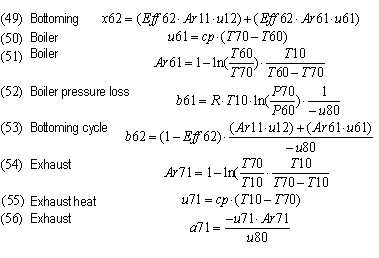17. EFFICIENCY OF TOTAL CYCLE     Because the efficiency of the cycle is calculated by subtracting all the separate bound energy B and helmholtz energy A losses from the helmholtz energy A supplied, different ones can be added or subtracted in Eq (59) to show the efficiency of the simple, combined, exchanged and cogeneration cycles.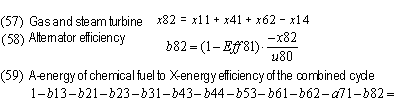18. REDUCTION OF COMBUSTION IRREVERSIBILITY'S (C17)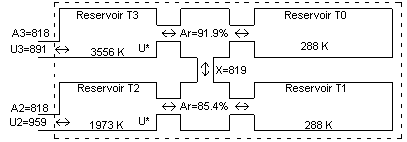Fig 10 Energy transformer model     It is known that electrochemical fuel cells can avoid combustion irreversibility's by converting chemical energy C directly into electricity. Real fuel cells though have considerable internal resistance and so produce thermal energy T as a by-product. Presently the best large fuel cell powerplants must use bottoming cycle engines to reach their A-X net electrical efficiency of 57% (Kordesch 1996) when operating at rated power. If the combustion irreversibility's are not considered, the Siemens V84.3A gas turbine combined cycle powerplant has an A-X net electrical efficiency of 85%. This indicates that the gas turbine type powerplant after the combustion process is inherently more efficient at converting helmholtz energy A than the fuel cell. Indeed Fig 8 shows that the A-X conversion efficiency of the compressor-expander portion of the gas turbine could approach 93% A-X efficiency in future high temperature gas turbine powerplants. Perhaps the fuel cell should be rather optimized as a gas turbine topping cycle. In the future it may be possible to design a very high temperature Solid Oxide fuel cell which would supply thermal energy to the Ceramic 4Pr gas turbine at up to 1973°K. A real powerplant A-X efficiency of up to 88% may be possible. 19. CONCLUSIONS     Future increases of efficiency of the gas turbine combined cycle are limited because of the low temperatures at the start of combustion. Recuperated gas turbines can be more efficient as the combustion inlet temperature is higher. The greatest loss of efficiency is produced in the combustion chamber and various methods could be employed in reducing these. 20. REFERENCES Adelman, S.T., Hofman, M.A., Baughn, J.W. Jan1995 A Methane-Steam Reformer for a Basically Chemically Recuperated Gas Turbine Journal of Engineering for Gas Turbines and Power ASME Press Ahern, J.E. 1980 The Exergy Method of Energy System Analysis, John Wiley & Sons Anheden, M., Nasholm, A., Svedberg, G. 1995 Chemical-looping Combustion-Efficient Conversion of Chemical Energy in Fuels Into Work, IECEC Paper CT-45 ASME Press Bailyn, M. 1994 A Survey of Thermodynamics, AIP Press NY USA Bolland, O. Apr1991 A Comparative Evaluation of Advanced Combined Cycle Alternatives, Journal of Engineering for Gas Turbines and Power, ASME Press El-Sayed, Y.M. Gaggioli, R.A. Mar1989 A Critical Review of Second Law Costing Methods-1 Background and Algebraic Procedures, Journal of Energy Resources Technology, ASME Press Horlock, J.H. Oct1992 The Rational Efficiency of Power Plants and Their Components, Journal of Engineering for Gas Turbines and Power, ASME Press El-Masri, M.A. 1987 A Modified, High-Efficiency, Recuperated Gas-Turbine Cycle 87-GT-13 ASME Press Kordesch, K., Simader, G. 1996 Fuel Cells and their Applications VCH Publishers NY, NY USA Moran, M.J., Sciubba, E. Apr1994 Exergy Analysis: Principles and Practice Journal of Engineering for Gas Turbines and Power, ASME Press Moran, M.J. 1982 Availability Analysis: A Guide to Efficient Energy Use, Prentice Hall NJ USA Wilson, D.G. 1984 The Design of High Efficiency Turbomachinery and Gas Turbines, The MIT Press, Cambridge Ma USA 21. SYMBOLS T0=ambient temperature °K, represents infinitely large heat sink T=absolute temperature °K of second reservoir of energy Cr=Carnot ratio Ar=Helmholtz ratio Gr=Gibbs ratio J=joules=1 Newton-meter of energy or j=joules/kg A, a, AE, ae=helmholtz energy kJ or kJ/kg B, b, BE, be=bound energy kJ or kJ/kg S, s=entropy kJ/kg-°K U, u, UE,ue=internal energy kJ or kJ/kg Q, q, QE, qe=caloric energy kJ or kJ/kg X, x, XE,xe=external energy kJ or kJ/kg V, v, VE, ve=expansion energy kJ, kJ/kg Y, y, YE,ye=clausius energy kJ or kJ/kg 10,11,20,30=points or processes By=air cooling bypass % of compressor c, cp=specific heat Eff=efficiency or effectiveness of process k=specific heat ratio L=pressure loss in bars n=gas coefficient of compression or expansion P, p=pressure, bars 1 atmosphere=1.010135 bar Pr=pressure ratio of gas turbine R=gas constant kJ/kg-K 22. NOTES  Read "Energy Science Made Simple" Chapter 11 for reasons why the hyphenated system is preferred by the author.  In this book the proposed units for temperature in the metric system are either degrees Celsius written °C or degrees Kelvin written °K. As of 1967 the presently accepted symbol for Kelvin is K. This however is confusing as Celsius is expressed in degrees and Kelvin is not. Also K can be mistaken for K, the proposed simple symbol for kinetic energy. Because the English alphabet has a limited amount of letters to be used as symbols, standard character set designations like the degree symbol can be used to further identify a unit. The degree symbol can easily be typed on a computer by holding down the Alt key while typing 248. 23. REVISION HISTORY 1999Apr10 First printing, 14 pages, 11 illustrations. 1999Apr19 Added missing paragraph formatting. Added bold wording. 1999Jun12 Changed symbol in Fig 4 from Cr to Ar. Added note about nonstandard °K symbol use. 1999Jul03 Corrected Fig 2,3,4 references, were unfortunately mixed up in converting to html document in Apr1999. 1999Sep22 Changed symbols X* and U* to V and Q. 2001Mar24 Changed to new hyphenated energy chart with SE now representing solution energy instead of subnuclear energy. 2002May03 Changed name from hyphenated energy system to plural energy system and energy-exergy system to singular energy system and made numerous minor changes. 2008Aug11 Changed some energy terms and deleted small subsection in chapter on combustion irreversibilities. COPYRIGHT © 1999-2020 All rights reserved Ben Wiens Energy Science Inc. Metro Vancouver BC Canada